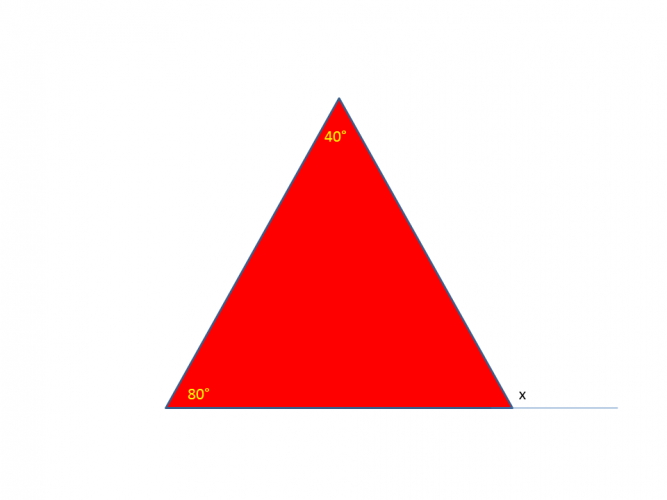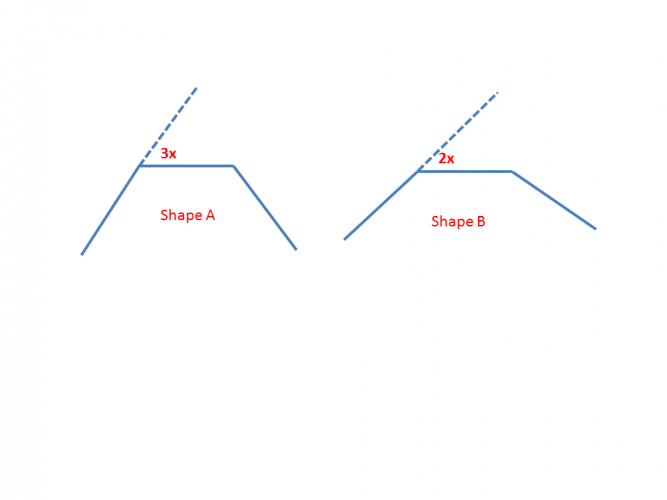# Calculate External Angles of a Polygon

In this worksheet, students will calculate the external angles of a polygon.Key stage:  KS 4

GCSE Subjects:   Maths

GCSE Boards:   AQA, Eduqas, Pearson Edexcel, OCR

Curriculum topic:   Geometry and Measures, Basic Geometry

Curriculum subtopic:   Properties and Constructions, Angles

Difficulty level:### QUESTION 1 of 10Lost again? Not to worry the GPS on your phone can help you find your way.

GPS uses angles, including exterior angles to help with the directions.  The mathematics is quite complex but has to start with the basics like we are going to here.

Exterior angles all you need to know for now anyway.Because the exterior angles of a triangle will fit around a point, and we know that angles around a point add up to 360°

We just use this along with the number of sides of the polygon to find the exterior angle.

Hopefully now you won't get lost.Calculate the size of the exterior angle of this hexagon.

55°

40°

60°

65°55°

40°

60°

65°Calculate the exterior angle of this shape.Can you help me?  I need to find the exterior angle of a polygon.

How do I do this?

 Divide the number of sides by 360° Multiply the number of sides by 360° Divide 360 by the number of sides To find the exterior angle of any polygonWhat is the exterior angle of a 20 sided shape?

 Divide the number of sides by 360° Multiply the number of sides by 360° Divide 360 by the number of sides To find the exterior angle of any polygonIf a shape has an exterior angle of 24° how many sides does it have?

Match up time.  Find the number of sides of a regular polygon with these exterior angles.

## Column B

10°
60
72
24
15°
36Find the value of xShape A is a 12 sided shape with an exterior angle of 3x.

The exterior angle of shape B is 2x.

 36° 12° 24° 12 16 15 18 The value of x is the number of sides shape B has isWhat is the exterior angle of an 11 sided shape?

• Question 1Calculate the size of the exterior angle of this hexagon.

40°
EDDIE SAYS
A hexagon has six sides so all we do is 360 ÷ 6 = 60°
• Question 2EDDIE SAYS
The exterior angle of this nonagon is 40° because all we do is 360 ÷ 9 = 40° Are you navigating these activities well?
• Question 3Calculate the exterior angle of this shape.

36
EDDIE SAYS
Okay so you weren't given the number of sides. No worries, just count them. This is a decagon, a 10 sided shape , so to calculate the exterior angle 360 ÷ 10 = 36°
• Question 4Can you help me?  I need to find the exterior angle of a polygon.

How do I do this?

 Divide the number of sides by 360° Multiply the number of sides by 360° Divide 360 by the number of sides To find the exterior angle of any polygon
EDDIE SAYS
It is quite simple really isn't it. Make sure you get the calculation the correct way around, otherwise you will get some weird and wonderful answers.
• Question 5What is the exterior angle of a 20 sided shape?

EDDIE SAYS
Bring it on. It doesn't matter about the number of sides does it. 360 ÷ 20 = 18°. We use 360 a lot in maths to help solve problems, a useful number don't you think.
• Question 6If a shape has an exterior angle of 24° how many sides does it have?

15
EDDIE SAYS
You know how to find the exterior angle by taking 360 and dividing by the number of sides. So to find the number of sides take 360 and divide by the angle given and hey presto. 360 ÷ 24 = 15 sides
• Question 7

Match up time.  Find the number of sides of a regular polygon with these exterior angles.

## Column B

10°
36
60
72
15°
24
EDDIE SAYS
External angles or number of sides no bother. What a great little formula to know. 360 ÷ by the exterior angle given = number of sides.
• Question 8Find the value of x

120
EDDIE SAYS
Sometimes a question presented in this way can make things look more complicated that it is. It is just the same. 360 & divide; 3 = 120° Can you see the connection here? The external angle of a triangle is the same as the sum of the two interior opposite angles.
• Question 9Shape A is a 12 sided shape with an exterior angle of 3x.

The exterior angle of shape B is 2x.

 36° 12° 24° 12 16 15 18 The value of x is the number of sides shape B has is
EDDIE SAYS
Oh text books and exam papers love these type of questions. But with your knowledge it is okay. Find the exterior angle of shape A first. 360 ÷ 10 = 36° ÷ 3 = 12 x = 12 Shape B the exterior angle = 2 x 12 = 24°: 360 ÷ 24 = 15 sides
• Question 10What is the exterior angle of an 11 sided shape?

32.7
EDDIE SAYS
Did you know that the name for an eleven sided shape is a hendecagon? Strange name don\'t you think. The size of the angle is 360 ÷ 11 = 32.7°
---- OR ----

Sign up for a £1 trial so you can track and measure your child's progress on this activity.

### What is EdPlace?

We're your National Curriculum aligned online education content provider helping each child succeed in English, maths and science from year 1 to GCSE. With an EdPlace account you’ll be able to track and measure progress, helping each child achieve their best. We build confidence and attainment by personalising each child’s learning at a level that suits them.

Get started